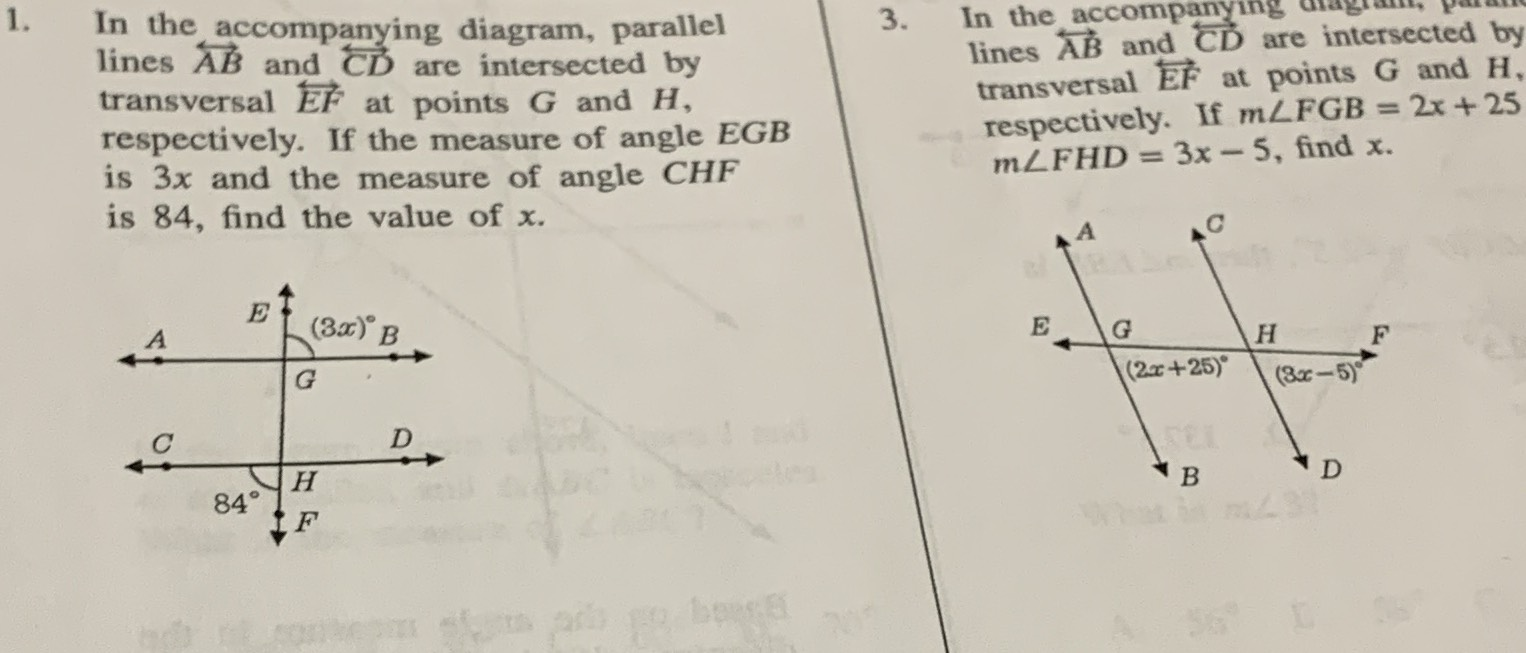### ¿Todavía tienes preguntas de matemáticas?

Pregunte a nuestros tutores expertos
Algebra
Pregunta1. In the accompanying diagram, parallel lines $$\overleftrightarrow { A B }$$ and $$\overleftrightarrow { C D }$$ are intersected by transversal $$\vec { E F }$$ at points $$G$$ and $$H$$ , respectively. If the measure of angle $$E G B$$ is $$3 x$$ and the measure of angle $$C H F$$ is $$84$$ , find the value of $$x$$ .